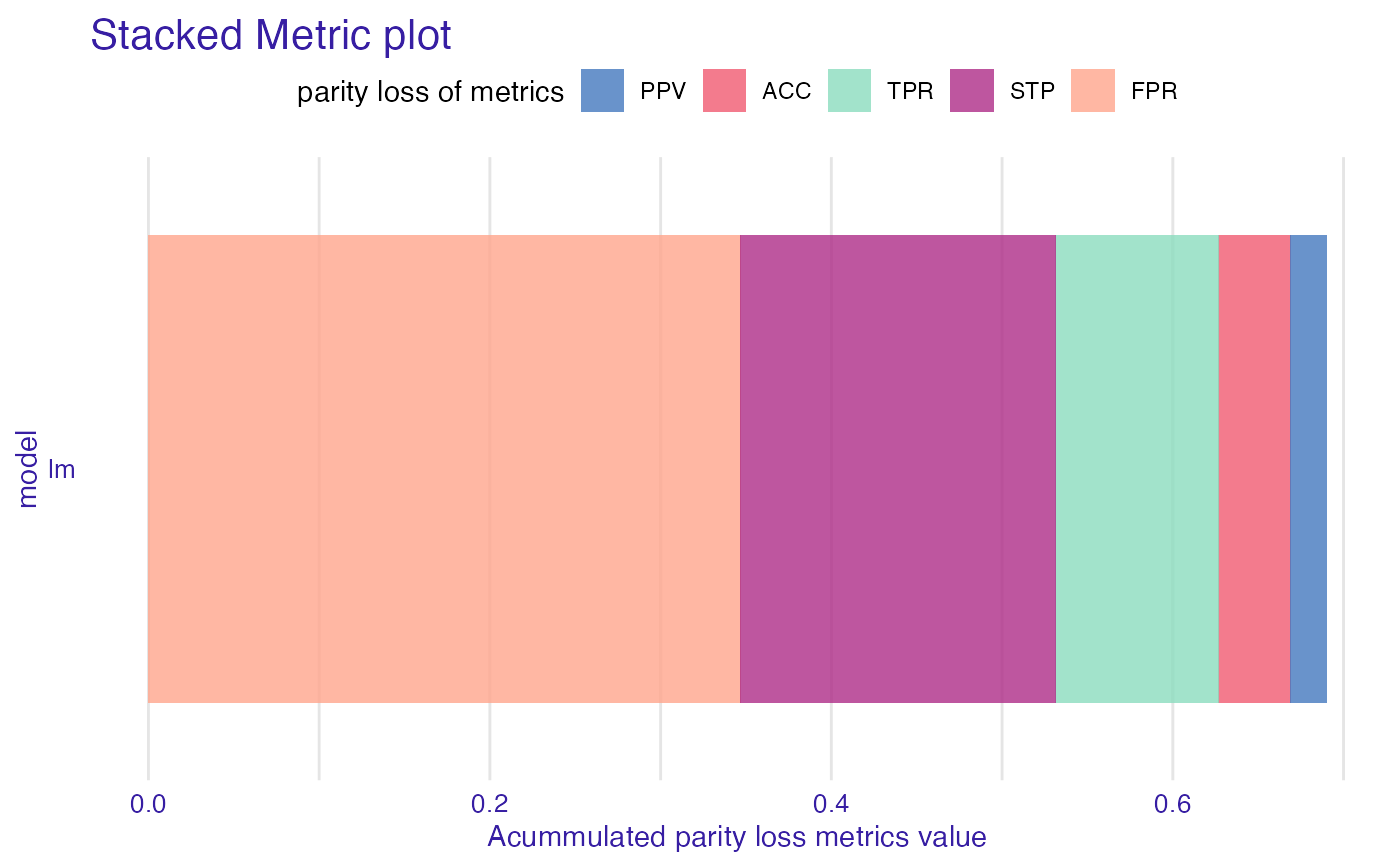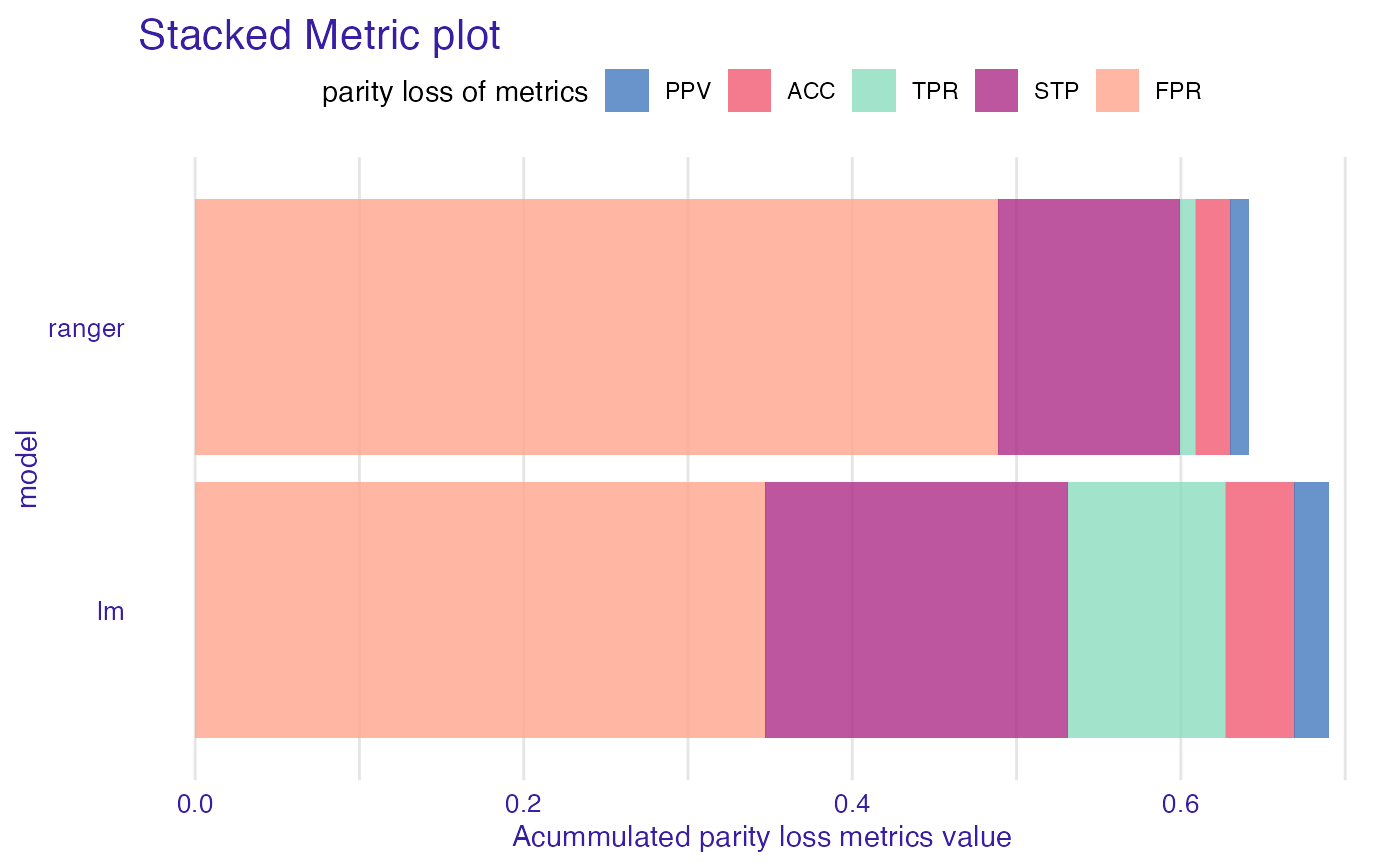Stack metrics sums parity loss metrics for all models. Higher value of stacked metrics means the model is less fair (has higher bias) for subgroups from protected vector.

stack_metrics(x, fairness_metrics = c("ACC", "TPR", "PPV", "FPR", "STP"))

## Arguments

x object of class fairness_object character, vector of fairness parity_loss metric names to include in plot. Full names are provided in fairess_check documentation.

## Value

stacked_metrics object. It contains data.frame with information about score for each metric and model.

## Examples


data("german")

y_numeric <- as.numeric(german$Risk) -1 lm_model <- glm(Risk~., data = german, family=binomial(link="logit")) explainer_lm <- DALEX::explain(lm_model, data = german[,-1], y = y_numeric) #> Preparation of a new explainer is initiated #> -> model label : lm ( default ) #> -> data : 1000 rows 9 cols #> -> target variable : 1000 values #> -> predict function : yhat.glm will be used ( default ) #> -> predicted values : No value for predict function target column. ( default ) #> -> model_info : package stats , ver. 4.1.0 , task classification ( default ) #> -> predicted values : numerical, min = 0.1369187 , mean = 0.7 , max = 0.9832426 #> -> residual function : difference between y and yhat ( default ) #> -> residuals : numerical, min = -0.9572803 , mean = 1.940006e-17 , max = 0.8283475 #> A new explainer has been created! fobject <- fairness_check(explainer_lm, protected = german$Sex,
privileged = "male")
#> Creating fairness classification object
#> -> Privileged subgroup		: character ( Ok  )
#> -> Protected variable		: factor ( Ok  )
#> -> Cutoff values for explainers	: 0.5 ( for all subgroups )
#> -> Fairness objects		: 0 objects
#> -> Checking explainers		: 1 in total (  compatible  )
#> -> Metric calculation		: 12/12 metrics calculated for all models
#>  Fairness object created succesfully

sm <- stack_metrics(fobject)
plot(sm)# \donttest{

rf_model <- ranger::ranger(Risk ~.,
data = german,
probability = TRUE,
num.trees = 200)

explainer_rf <- DALEX::explain(rf_model, data = german[,-1], y = y_numeric)
#> Preparation of a new explainer is initiated
#>   -> model label       :  ranger  (  default  )
#>   -> data              :  1000  rows  9  cols
#>   -> target variable   :  1000  values
#>   -> predict function  :  yhat.ranger  will be used (  default  )
#>   -> predicted values  :  No value for predict function target column. (  default  )
#>   -> model_info        :  package ranger , ver. 0.12.1 , task classification (  default  )
#>   -> predicted values  :  numerical, min =  0.0644623 , mean =  0.6963466 , max =  0.9982302
#>   -> residual function :  difference between y and yhat (  default  )
#>   -> residuals         :  numerical, min =  -0.7662778 , mean =  0.003653402 , max =  0.622853
#>   A new explainer has been created!

fobject <- fairness_check(explainer_rf, fobject)
#> Creating fairness classification object
#> -> Privileged subgroup		: character ( from first fairness object  )
#> -> Protected variable		: factor ( from first fairness object  )
#> -> Cutoff values for explainers	: 0.5 ( for all subgroups )
#> -> Fairness objects		: 1 object (  compatible  )
#> -> Checking explainers		: 2 in total (  compatible  )
#> -> Metric calculation		: 10/12 metrics calculated for all models ( 2 NA created )
#>  Fairness object created succesfully

sm <- stack_metrics(fobject)
plot(sm)# }